# How to Create A Model Builder in QGIS

Data processing is one of a main tasks in GIS. There are many tools available in a GIS software both proprietary or open source to do data processing. In a data processing workflow, commonly some steps are required. The steps that involved are connected one to another in a series from input to output. Therefore we can arrange and connect all processing steps in a model as in figure 1. In this tutorial we will learn how to create a model builder in QGIS which is called graphical modeller. I will go step by step from a simple to a complex example.Figure 1. QGIS Graphical Modeller

### Starting Graphical Modeller

To start a model in QGIS, we have to open a graphical modeller window from Processing menu then select Graphical Modeler as shown in figure 2. An empty graphical modeller window will be opened as in figure 3.Figure 2. Open Graphical Modeller in QGISFigure 3. QGIS Graphical Modeller Window

In the graphical modeller window, we can see some icons and menus. But firstly we will focus on the left window for Inputs and Algorithms section. Inputs are all input variables in a model such as: String, Boolean, Vector Layer, Raster Layer, Expression, etc. Algorithms are all tools that will be used to process input variables. In the process, an algorithm/tool will return an output that will be used for another tool till final output.

### Simple Model Example: Buffer a Vector Layer

Let's see a simple example. We will do a buffer for road segments as in figure 4. For this process, the input will be a vector layer and algorithm is buffer.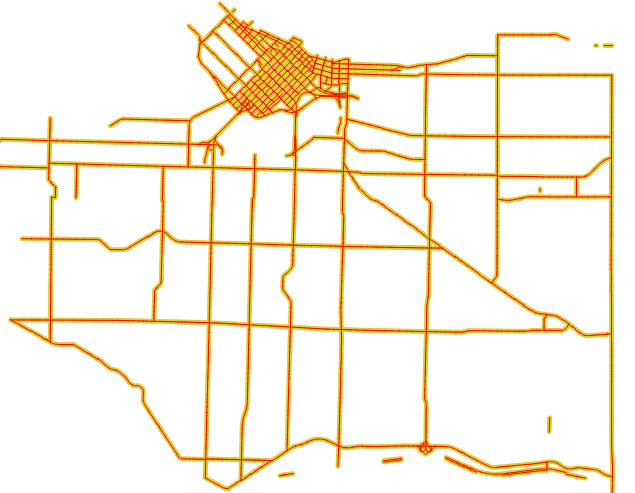Figure 4. Buffered road segments

Below are the steps to create a buffer model.

1. From the modeller window, select Inputs tab and then select Vector Layer by double click or drag and drop it into model canvas as in figure 5. The Input parameter definition window will appear as in figure 6. In this window we can customize some vector input parameters like description, geometry type and give a comment.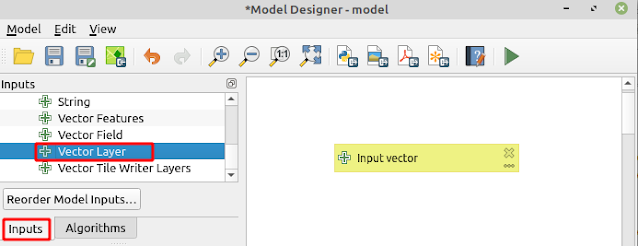Figure 5. Vector Layer InputFigure 6. Vector Layer Parameter Definition

2. Select Algorithms tab. There are numerous algorithms are grouped into a respective group. If you know where an algorithm is, go for it. If not, search for it from the searching toolbar. For this example I search for buffer algorithm as in figure 7. Add it into model canvas.Figure 7. Buffer Algorithm

3. The buffer algorithm window will appear as in figure 8. As usual here we can customize some parameters like distance, dissolve option, end cap style, etc. But  I want to emphasize on Input layer options. If you look at the figure, there are some options as explained below:

• Value: this option takes a pre-determined input value. It can be chosen from an existing layer that already added into QGIS map canvas or browse into a particular path when the model is running.
• Pre-calculated Value: this option takes input from an expression.
• Model Input: the input will be taken from an input variable through model canvas.
• Algorithm input: this option takes input from the output of an algorithm.

I want to get input from the Vector Layer that already added into model canvas. For that I select Model Input as input layer. Other parameter options are the same with the common buffer tool. Last parameter with green arrow icon (before Dependencies) is the output parameter, here can be specified a name for the final output. If it's not a final output leave it empty as a temporary output.Figure 8. Buffer algorithm parameter options

4. The final model is like figure 9. From the figure can be seen that the model has an input with one algorithm and an output. Before saving the model, give it a name in the Model Properties options.Figure 9. A simple buffer model

5. The model is ready to run. For a complex model, I suggest to validate the model to inspect error(s) in the model. For validating the model can be done using Validate Model tool that can be found in the Model menu as shown in figure 10. Finally run the model using F5 key or select Run Model menu or  by pushing green play button.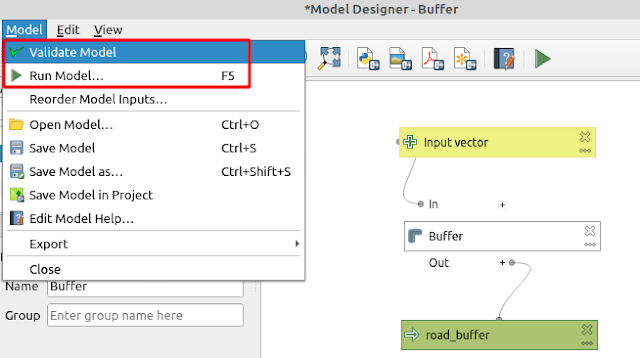Figure 10. Validate and Run model

### Generating Contour Model

From the first example, I hope you can grasp some basic concepts about creating a model with graphical modeller in QGIS. To enhance the knowledge, let's create another model to generate contour lines from Digital Elevation Model (DEM) data. Step by step process to generate contour lines from DEM is explained in this tutorial (How to create contour lines in QGIS). I suggest to check it out, to get an idea how to do it step by step without a model. Furthermore when building a model, understanding all processes that involved in the model is the key.

The model consist of two inputs and two algorithms. Below is the summary for the model. The final model can be seen as in figure 11.

• Input: Raster Layer(Input DEM), Number(Contour Interval)
• Algorithm: Contour, Smooth
• Output: Contour linesFIgure 11. Contour generating model

The steps to build the model as follow.

1. From Inputs tab select Raster Layer and Number. For raster layer give Input DEM as description and Contour interval for Number.

2. Switch to Algorithms, search for Contour, then select the GDAL Contour tool.  The contour parameter options will appear as in figure 12. Select Model Input For input layer and choose Input DEM. The same with Interval between contour lines parameter select Contour Interval.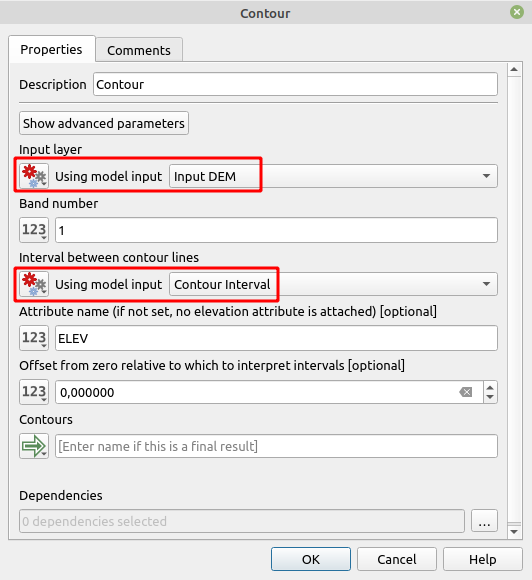Figure 12. Contour algorithm parameter options

3. Next, we will do smoothing (If you read step by step tutorial, from the link above, you will know why we do this process). The Smooth tool can be found in the Vector Geometry group tool. Figure 13 shows the smooth parameter options. In the Input layer select Using algorithm output, because it will process the output from Contour algorithm. Next for the output give it a name contour lines.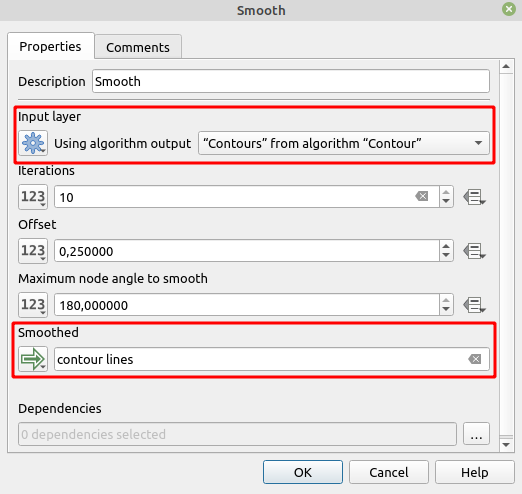Figure 13. Smooth algorithm parameter options

4. The final model is shown like figure 11. Validate and run the model. The model window will appear as in figure 14. If you look at closely the Contour model Graphical User Interface (GUI), the Contour interval input is on the top. I think  it's a little bit weird, cause usually the file/data input comes first. So Input DEM parameter should be on the top. To change the order can be used Reorder Model Inputs from the Model menu. Figure 15 shows the Reorder model inputs window. Through this window the order of inputs model can be adjusted by moving up/down.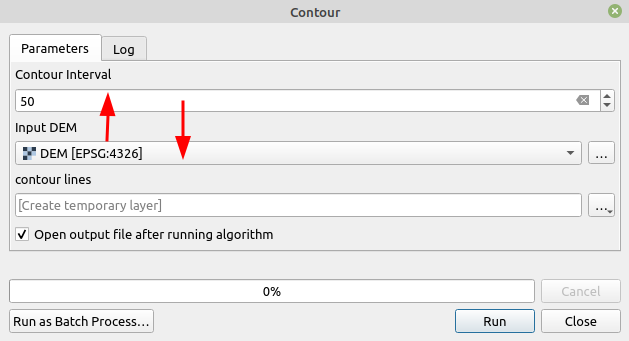Figure 14. Contour model GUI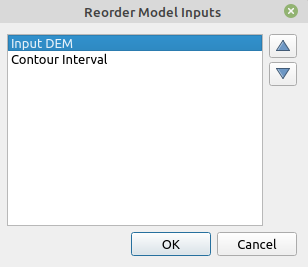Figure 15. Reorder model inputs

To create contour lines using this model, a DEM data is required as input. If you don't have one, check out this tutorial How to download DEM with QGIS.

### NDVI and EVI Processing Model

In this example, we will build a little complex model to calculate Normalized Difference Vegetation Index (NDVI) and Enhanced Vegetation Index (EVI). The main idea of this model is to compute NDVI or EVI based on a user selection, input all required satellite imagery, clip into interest area, perform NDVI/EVI calculation and convert the result to polygon if a user want it.

Following is summary for this model.

• Input: Satellite imagery(NIR, RED and Blue band), Masking Polygon, Enumerator for selecting NDVI or EVI, Boolean to check the result conversion into polygon.
• Algorithm: Clip, Raster calculator, Polygonize(raster to polygon)
• Ouput: Raster(NDVI/EVI), polygon.

1. From the Inputs select Add Raster Layer for each required bands.

2. Add a Vector Layer as a mask polygon to clip the raster bands.

3. Switch to Algorithms tab, add Clip raster by mask layer to clip each raster. The input for this tool is model input for each raster band and mask polygon. The model canvas up to this step is shown like figure 16.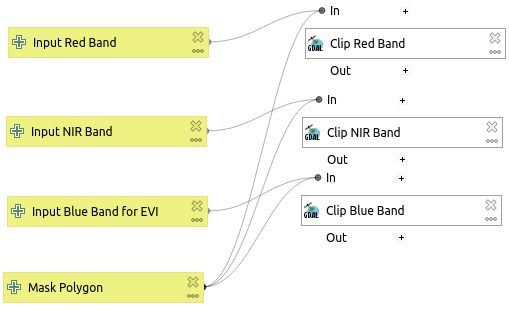Figure 16. Inputs and clip algorithm

4. From Inputs, add Enumerator. Then add two items NDVI and EVI as in figure 17.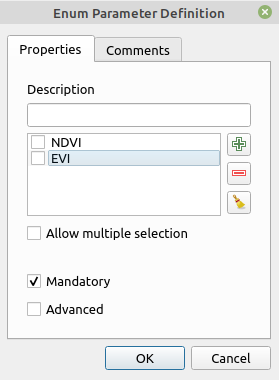Figure 17. Enumerator input

5. Add Boolean input to provide an option for user to convert NDVI/EVI to polygon as in figure 18.Figure 18. Boolean Inputs

6. Before proceeding to the next algorithm. In this step we are creating a conditional options. From Algorithms, select Conditional branch in Modeler tools. Create four conditions as seen in figure 19. Condition 1 and 2 are for enumerator input selection, which return the selected item index 0 or 1. Condition 3 and 4 are for boolean options, to convert raster output to polygon. The boolean conditions return True if checked otherwise False.Figure 19. Conditional branch

6. Add GDAL Raster calculator algorithm. The GDAL Raster calculator defines each input in A,B,C,....layer as shown in figure 19. Because the inputs are clipped band output, then the input type is Using algorithm output. Figure 20 is the raster calculator window to calculate EVI. Therefore three inputs are required, Red, NIR and Blue Band for respective A, B and C.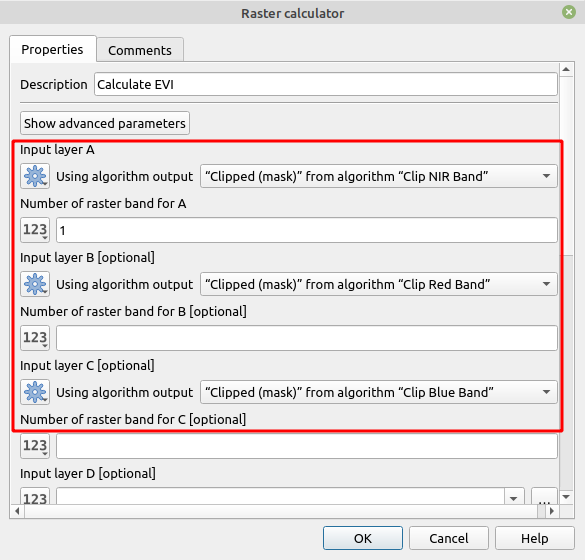Figure 20. GDAL Raster calculator

7. In the calculation field fill EVI formula as in figure 21. Then determine an output name in the Calculated field. Lastly before closing this window, click the Dependencies button. Here we have to define a condition. When the condition is passed, the algorithm will be executed.Figure 21. Raster calculator window

Figure 22 shows the conditions in the model. In the figure can be seen that the condition "EVI" from algorithm "Conditional branch" is checked, which means the algorithm will be executed if EVI item in numerator input is selected.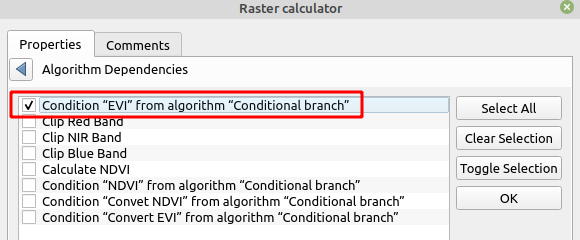Figure 22. Dependencies conditions

The same procedures area applied for NDVI raster calculation, but with different condition ("NDVI" from algorithm "Conditional branch"). The updated model up to this step can be seen as in figure 23.Figure 23. Updated NDVI-EVI model

Before proceeding to the next step, I strongly suggest to run the model to see if it works fine as expected. If the model works fine, it will produce NDVI and EVI in a raster format.

8. This step will convert the output of NDVI or EVI in raster format into vector format in polygon geometry. For that, search a GDAL algorithm which is called Polygonize. Figure 24 shows the Polygonize window to convert NDVI to vector polygon. In the Input layer, select Using algorithm output option with "Calculated" from algorithm "Calculate NDVI" as selected value. In the Vectorized field, give a name for the vector output. And last one for Dependencies option check Condition "Convert NDVI" from algorithm "Calculated branch". It means, when boolean condition to convert NDVI to polygon is checked (True), this algorithm will be executed.Figure 24. Polygonize algorithm

The same procedure applies to EVI polygonize algorithm. NDVI/EVI raster to vector conversion in the last processing step in this model. The final model can be seen in figure 25. Download the model.Figure 25. NDVI-EVI final model

Finally, it's time to run the model. Figure 26 shows the model GUI. The model in action can be seen in the figure 27. The running model in figure 27 is using Sentinel satellite imagery for Red, NIR and Blue band. Visit this tutorial to download Sentinel satellite imagery in QGIS.Figure 26. NDVI-EVI Model GUIFigure 27. NDVI-EVI model is running

That's all this tutorial how to build a processing model builder in QGIS. In this tutorial we learn how to start a graphical modeller, build a simple model and complex model. This model builder capability in QGIS is really helpful to do a complex data processing task or automate repetitive processing task which can save a lot of time. Hopefully it's useful for you and thanks for reading.